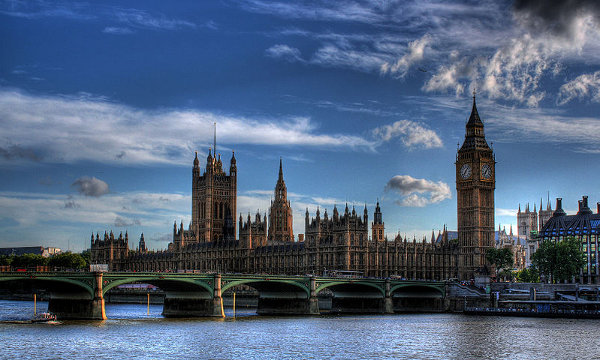# Complementary Events

A Complementary Event is when the event will not happen.

The sum of probabilities of an event and its complementary event add up to 1.

Therefore, to find the probability of a complementary event, subtract the probability of the event from 1.

## Example One - Probability of Today is NOT My Birthday

The chance that any particular is someone's birthday is 1 out of 365. What is the probability that today is not my birthday?

Probability (Birthday) = 1365 = 0.0027
Probability (Not Birthday) = 1 – 0.0027 = 0.9973

## Example Two - Probability of NOT Raining in EnglandIt rains about 200 days each year in England. What is the chance that it will not rain on any given day in England?

Probability (Rain) = 200365 = 0.55
Probability (Not Rain) = 1 – 0.55 = 0.45

## Example Three - Probability of NOT Throwing Snake-Eyes

"Snake-eyes" is double ones when throwing two die (because the two dots look like two small eyes). The probability of throwing 1 and 1 on two die is 1 out of 36. What is the probability that I will not throw snake-eyes?

Probability (Double Ones) = 136 = 0.028
Probability (Not Double Ones) = 1 – 0.028 = 0.972

## Questions

 RISKY BUSINESS STORIES PROBABILITY OF THIS EVENT HAPPENING Q1. Honduras in South America has almost the highest homicide rate in the world. There are 78 murders for every 100 000 people. Probability (Murdered in Honduras in a year)= 78 murders ÷ 100000 people= 0.00078 Q2. The University of North Texas Health Science Center states that there were 16141 fatalities in car accidents in USA from 2001 to 2007 caused by texting while driving. Assume that the USA population is 307 million. Probability (Death while texting and driving in a year)= (16141 deaths ÷ 6 yr) ÷ 307 000 000 people= 0.0000088 Q3. According to the US National Weather Service, there are 8640000 lightning strikes per day on Earth. Assume the Earth's area is 510 million square kilometres. Probability (Hit by lightning in a year)= 8640000 strikes ÷ 510 000 000 km2= 0.017 Q4. The US National Library of Medicine states that the highest reported number of Salmonella food poisoning cases in 2003 was in Bulgaria with 2741 cases per 100 thousand people. Probability (Food poisoning in Bulgaria in a year)= 2741 cases ÷ 100 000 people= 0.027

Find the probability of the complementary event (that it will not happen) of each of the risky business stories in the table above.